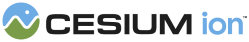#EllipsoidGeometryUpdater

#### new Cesium.EllipsoidGeometryUpdater(entity, scene) DataSources/EllipsoidGeometryUpdater.js 62

A `GeometryUpdater` for ellipsoids. Clients do not normally create this class directly, but instead rely on `DataSourceDisplay`.
Name Type Description
`entity` Entity The entity containing the geometry to be visualized.
`scene` Scene The scene where visualization is taking place.

### Methods

#### createFillGeometryInstance(time, skipModelMatrix, modelMatrixResult) → GeometryInstance DataSources/EllipsoidGeometryUpdater.js 114

Creates the geometry instance which represents the fill of the geometry.
Name Type Default Description
`time` JulianDate The time to use when retrieving initial attribute values.
`skipModelMatrix` Boolean `false` optional Whether to compute a model matrix for the geometry instance
`modelMatrixResult` Matrix4 optional Used to store the result of the model matrix calculation
##### Returns:
The geometry instance representing the filled portion of the geometry.
##### Throws:
• DeveloperError : This instance does not represent a filled geometry.

#### createOutlineGeometryInstance(time, skipModelMatrix, modelMatrixResult) → GeometryInstance DataSources/EllipsoidGeometryUpdater.js 198

Creates the geometry instance which represents the outline of the geometry.
Name Type Default Description
`time` JulianDate The time to use when retrieving initial attribute values.
`skipModelMatrix` Boolean `false` optional Whether to compute a model matrix for the geometry instance
`modelMatrixResult` Matrix4 optional Used to store the result of the model matrix calculation
##### Returns:
The geometry instance representing the outline portion of the geometry.
##### Throws:
• DeveloperError : This instance does not represent an outlined geometry.
Need help? The fastest way to get answers is from the community and team on the Cesium Forum.